## Main

The Leidenfrost effect is commonly observed in the kitchen—splash a droplet of water onto a hot pan and, rather than boiling, it counterintuitively floats above the surface1. Far beyond a curiosity, this effect plays a critical role in industrial settings ranging from alloy production plants4 to nuclear reactors19 and provides a mechanism to reduce drag in fluid4,6 and solid18 transport. Although first described more than two centuries ago, issues as fundamental as droplet shape12,13, the dynamics during impact8,10,20, and the effects of substrate texturing3,7,11,15,16 are only recently becoming understood. One issue that has remained unquestioned is the potential importance of the mechanical properties of the object itself. For sublimable solids such as dry ice, the Young’s modulus is far too large (10 GPa) for mechanical deformations to be relevant15,16,17. In liquids, surface tension can lead to quasi-elasticity for tiny droplets14, but otherwise its influence is limited to capillary oscillations13.

Here we introduce a new type of Leidenfrost effect that occurs with vaporizable soft solids—in our experiments, water-saturated hydrogel spheres (diameters 1.49 ± 0.01 cm, masses 1.75 ± 0.03 g). Despite consisting of 99% water, these behave like linear elastic solids (Young’s moduli Y = 50 ± 4 kPa; see the Methods and Supplementary Fig. 2). The effect is illustrated in Fig. 1a, where we show top-down tracks of five dyed hydrogel spheres cast onto a ceramic-coated aluminium surface at 215 °C. Immediately on contact the spheres exhibit energetic activation, frenetically travelling around the surface at speeds of up to 0.5 m s−1 and emitting high-pitched screeching noises (see Supplementary Movie 1). This demonstrates the potential usefulness of the effect as an energy injection strategy, particularly to create macroscopic active matter21,22. While the tracks convey horizontal motion, this is achieved through sustained vertical bouncing where the spheres repeatedly reach heights of 3–4 cm. The effect is long-lived—a sphere typically bounces for two to three minutes (103 bounces), and occasionally we observe lifetimes up to ten minutes. The activity would continue longer if the hydrogel material itself were tougher—the cessation of motion is invariably associated with fracture (Fig. 1b).Figure 1: Sustained bouncing of hydrogel spheres on a hot surface.

With side-view video of a single sphere bouncing on a gently curved plate (see set-up of Fig. 1c), we isolate the vertical motion (Fig. 1d–f and Supplementary Movie 2). For a drop height of 6 cm onto a ‘cold’ (25 °C) surface, the sphere behaves like an inelastic ball, losing energy during each impact and quickly coming to rest (Fig. 1d). With the same drop height and a ‘hot’ (215 °C) surface (Fig. 1e), the sphere loses energy initially, but soon reaches a steady bounce height of a few centimetres. Spheres dropped from below this height climb higher with every bounce—ultimately up to the same steady state (Fig. 1f). Simultaneous plots of the vertical trajectories and audio traces show that the screeching occurs only in the hot experiments and coincides with each impact (Fig. 2a, b).

The existence of a steady bounce height indicates that spheres in the hot experiments gain kinetic energy during their interaction with the surface. To quantify this, we first analyse the cold experiments (inset Fig. 2a) and determine the rebound curve (Hi+1 versus Hi). By subtracting the anticipated ‘cold’ rebound height from the measured one in the hot experiments, we determine the kinetic energy injected during impact with the hot surface (see Methods for full details). For steady-state bouncing, this amounts to around 102 μJ (6 mm in added bounce height), although with significant bounce-to-bounce fluctuations (inset Fig. 2b). By performing drops over a range of heights with an ensemble of similar spheres we obtain the average energy injection versus drop height (Fig. 2c). Plotting the energy injection and loss curves on the same graph produces an intersection point at approximately 3.5 cm, that is, the steady bounce height.

One naturally suspects this behaviour is linked to vaporization of the water-saturated gel. By measuring the mass lost by spheres versus how long they bounce on the hot surface (Fig. 2d) we verify this—on average, they boil 1.5 × 102 μg per impact. How does the vaporization process unfold? Focusing on a single impact at significantly higher spatial and temporal resolution, we discover complex dynamics at the sphere/substrate interface. The image sequence in Fig. 3a shows that throughout the total duration (8 ms) of a single impact, a minute gap repeatedly opens and closes below the sphere at a much faster timescale. This agitation is best appreciated in Supplementary Movies 3 and 4, which further reveal that each oscillation launches a Rayleigh wave that propagates around the sphere’s surface. Using the central region of the video, we see that the gap reaches heights of 102 μm before throttling back to the surface. The gap power spectrum (Fig. 3c) has clear peaks near 2–3 kHz. These peaks are also present in the audio spectrum, which unveils the oscillations as the source of the audible screeching.

These observations are starkly different from the equilibrium Leidenfrost effect, where the stable (and silent) gap is governed by a delicate balance between vaporization, viscous squeeze flow, and the object’s weight2,5. Recent experiments with liquid droplets impinging on hot surfaces10,20 show that for sufficiently high impact velocities the vapour layer is squeezed out and the droplet makes physical contact with the substrate, leading to accelerated vaporization and a barrage of bubbles that tear upwards through the liquid. For our impacting spheres, we also expect physical contact and accelerated vaporization, but the integrity of the solid gel precludes the nucleation and escape of bubbles through the inside of the material—instead, vaporization is confined to the gel–substrate interface. Furthermore, whereas liquids store no elastic energy, and stiff solids such as dry ice barely deform at all, the spheres in our experiments are solid yet soft, which means that energy stored in pressurized vapour can be converted into mechanical energy through elastic deformation.

On the basis of these considerations, we now propose a picture for the underlying physics that recasts each gap oscillation as a thermodynamic cycle that does mechanical work on the sphere (Fig. 4a). The first stage of the cycle commences each time the sphere bottom comes into physical contact with the surface. This causes rapid vaporization, but the localization of the generated vapour at the interface effectively traps it in a ‘pocket’ (as illustrated for stage I of Fig. 4a). The growing pressure from this vapour deforms the sphere’s underbelly and causes the volume of the pocket to expand until its radius reaches the edge of the Hertzian contact and its height reaches a value l. Now the visible gap opens up and stage II begins where the vapour is blown out by the overpressure. Once the pressure is sufficiently reduced, stage III begins during which the sphere bottom elastically recoils toward the substrate, thus reinitiating stage I. The asymmetry of the pressure evolution on the upward/downward strokes of this cycle renders the area enclosed in the pressure–volume diagram greater than zero, which results in an increase in the sphere’s mechanical energy. Remarkably, this energy injection is achieved with the fuel (water), mechanism (gap oscillations) and mechanical output (increased mechanical energy) embedded in a single soft material—the sphere is effectively a soft engine that harvests energy from the hot surface.

Characterizing the physics behind this engine from first principles involves a complex interplay of vaporization, compressed gas dynamics, and mechanical deformation. We now lay out a simplified numerical model that couples these three ingredients and highlights the essential physics of this elastic Leidenfrost effect. Complete details are included in the Methods, where we also present calculations based on our experiments to predict the appropriate simulation parameters. As illustrated in Fig. 4b, we mimic the soft sphere with a one-dimensional chain of N identical masses (mass m) connected by N − 1 identical springs (rest length δ, stiffness κ). Simulating a chain does not reproduce the geometric nonlinearities associated with a Hertzian contact23. However, it enables us to resolve elastic deformations at the interface (that is, the gap oscillations) independently from the centre-of-mass motion of the impacting object, which is a critical aspect of the observed phenomena. (We further clarify this issue in the Supplementary Text.) We solve for the dynamics of this chain as it impacts into a hard surface by specifying the forces and initial conditions for each mass and numerically integrating the equations of motion. Denoting the index of the bottom mass as i = 0 and counting upwards, these are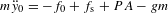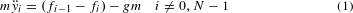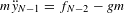Here g is the acceleration due to gravity, fi is the compressive force in the spring between masses i and i + 1, fs is the force provided by the hard surface, P is the pressure from the vapour and A is the instantaneous contact area. For both ‘cold’ and ‘hot’ impacts, we model the force provided by the hard surface, fs, as a stiff spring that acts on the bottommost mass once it passes y = 0.

Impacts onto a ‘hot’ surface include the additional force that arises from the vapour pressure, P, that develops below the bottommost mass. To reproduce the periodic pressure trapping, we initialize the pressure to P = 0 Pa and co-evolve it as follows. We approximate the build-up that occurs each time the bottommost mass reaches the surface (stage I) with linear growth (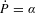). For the escape that occurs once the gap has opened up (stages II and III), we approximate the vapour release with exponential decay (). Consistent with our preceding discussion, we demarcate the transition from pressure growth to decay each time the bottommost mass rises above the lengthscale l. The pressure acts over an area, A, that evolves throughout the impact according to the overlap of an imaginary sphere located at the centre of mass of the chain with the surface at y = 0.

Despite our model’s simplicity, the simulations qualitatively and semi-quantitatively capture all of the experimental observations (for qualitative comparison, see Supplementary Movie 5). During impact, the position of the lowest mass in the chain, that is, the gap, rises up to heights on the order of 102 μm with a frequency around 2.5 kHz, thus reproducing the observed oscillations (Fig. 4c). Calculating the kinetic energy injection and loss curves exactly as in the experiments, we see an intersection point at drop heights of a few centimetres and a steady-state kinetic energy injection on the order of 102 μJ. With the mechanism laid out explicitly, we can estimate an upper bound for the total energy injection during an equilibrium bounce as Etotal FavlNosc 5 × 102 μJ (where Nosc 10 is the number of gap oscillations and Fav 0.5 N is the average impact loading—see Methods and Supplementary Fig. 3). This value exceeds the measured kinetic energy injection of Fig. 2 (102 μJ) and is consistent with the fact that energy is also pumped into internal vibrations, that is, the Rayleigh waves and screeching.

We have introduced a new type of Leidenfrost effect that occurs with vaporizable soft solids. Beyond the gentle hovering observed with liquids and stiff materials, soft solids are capable of energetic activation in the form of sustained bouncing. Our experiments and numerical simulations reveal that the mechanism behind this behaviour is the coupling between vapour release and elastic deformations, which leads to microscopic work cycles at the sphere/substrate interface that inject mechanical energy. In addition to this fundamental result, our findings provide a tool for activating hydrogels in other fields. As a concrete example, Supplementary Movie 1 illustrates that studying collective phenomena in systems of ‘active bouncers’ is already within reach. Given the increasing use of hydrogels in soft robotics24 and microfluidics25,26, it is conceivable that embedding heating elements in those systems could lead to useful actuation there, too—particularly given that techniques to rapidly fabricate hydrogels27,28, bond them to diverse surfaces29, and increase their toughness30 are steadily advancing. Finally, although the system we have presented harvests energy from a heat reservoir, we imagine that other energy sources, for example, vapour blown through a porous plate or a chemically active surface, could produce a similar effect. The elastic Leidenfrost effect therefore offers a template on how elastic deformations can be leveraged to create energetic activation of soft materials.

## Methods

### Sphere preparation.

We prepared commercially available hydrogel spheres (Educational Innovations GB-710) by adding dehydrated specimens to a mildly saline solution (0.6 g NaCl/KCl table salt per 1.0 l Milli-Q water). As shown in Supplementary Fig. 1, the distribution (mean and spread) of masses of the dehydrated spheres is 24 ± 1 mg, while for the swollen spheres it is 1.75 ± 0.13 g. The water content by mass is therefore 98.6 ± 0.1%. Given that the Young’s moduli change quickly with sphere size (Supplementary Fig. 2), we performed experiments with a subset of spheres that had a distribution M = 1.75 ± 0.03 g. For imaging data, we dyed the otherwise clear spheres with food colouring (Rainbow Dust ProGel).

### Surfaces.

For the data in Figs 1d–f and 2a, b and the energy loss measurements in Fig. 2c, we used an aluminium surface with a gentle spherical curvature (radius 81.9 cm) to keep the sphere within the field of view. In cold experiments we applied a superhydrophobic coating (Glaco Mirror Coat Zero) to mitigate wetting. Vaporization prevented wetting in hot experiments. The aluminium surface permitted observation of sequential impacts, but it easily became sullied. This made subsequent interactions erratic and required frequent cool-down so it could be cleaned. For the energy injection measurements of Fig. 2c, we used a flat, ceramic-coated aluminium surface. This permitted us to observe only a few bounces at a time, but allowed us to clean the surface while hot and avoid cool-down. The roughness of both the flat and curved surfaces was less than 5 μm. We heated the surfaces with a hotplate (Stuart US150 Hot Stirrer, 700 W) and measured their temperatures to within ±5 °C with a thermocouple.

### Sphere Young’s modulus.

We characterized the spheres’ mechanical properties using an Instron (model 5965) equipped with a 10 N load cell to take force-displacement curves for individual specimens sandwiched between two vertical cross heads (Supplementary Fig. 2a). We attached fine (1200 grit) sandpaper to the cross heads to prevent slippage. We coated the sandpaper with superhydrophobic spray (Glaco Mirror Coat Zero) to mitigate wetting. The Young’s modulus was calculated by fitting the force-displacement curve to the equation for a Hertzian sphere compressed between two hard half-spaces31, that is,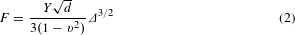Here Y is the Young’s modulus, d is the diameter, ν is the Poisson’s ratio (v = 0.5) and Δ is the cross-head displacement. The value of the Young’s modulus varies from sphere to sphere and with the diameter (Supplementary Fig. 2b). For the spheres we used in the experiments, the distribution of Young’s moduli is Y = 50 ± 4 kPa.

### Bounce heights and trajectory analysis.

Our experimental set-up provided a variety of ways to measure a sphere’s vertical trajectory and bounce height. While the most straightforward would seem to be with the camera, this has the disadvantages of: poorly resolving small drop heights; and requiring inconveniently large amounts of data and analysis. Instead, we used the force sensors to define the contact intervals and backed out the vertical trajectories from Newton’s laws23,32,33,34 (Supplementary Fig. 3a). We take tiout to be the time when impact i ends and ti+1in to be the time when impact i + 1 begins and furthermore define Δt = ti+1intiout and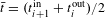. The maximum height in the parabolic flight between is Hi+1 = gΔt2/8, where g = 9.8 m s−2. The trajectory is given by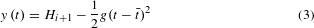This is valid as long as aerodynamic drag is small compared with the sphere’s weight. For spheres with a diameter of 1.5 cm moving through air at 1.0 m s−1 (the maximum velocity in any of our experiments), the drag term is on the order of ρairπd2V2/4 1 × 10−4 N—two orders of magnitude smaller than the weight.

For impacts on the hot surface we modified this procedure on account of the gap oscillations, which modulate the force sensor signal at high frequency. Additionally, the sensor picks up other spurious oscillations ranging from 1–10 kHz due to mechanical resonances in our set-up. We therefore quantified the response of an impact onto a hot surface by first performing a low-pass (<1 kHz) filter and then defining tc and Fmax the same way as in the cold experiments (Supplementary Fig. 3b). The gross features of impact involve low enough frequencies (<500 Hz) to be preserved.

### Energy injection.

We measured the kinetic energy injection by comparing the rebound heights for spheres dropped on cold and hot surfaces. The bouncing on a cold surface is close to what would be expected for impacts with a constant coefficient of restitution, which would give Hi+1 = ɛ2Hi. However, ɛ deviates from constant behaviour at low and especially at high Hi (Supplementary Fig. 4). To account for this, we binned our data and constructed an interpolated coefficient of restitution curve for the cold surface, ɛ(Hi), as shown in Supplementary Fig. 4b. The energy injection is then given by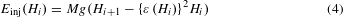We remark that the form of the curve, ɛ(Hi), is not consistent with dissipation from plastic deformation23 or viscoelasticity35. This reveals that the energy lost during impact is mainly transferred to spheroidal oscillations36, which are clearly visible in Supplementary Movie 3. These oscillations damp out over the long parabolic flights between impacts. Note these spheroidal oscillations are large wavelength (λ d) and are not the same as the short-wavelength Rayleigh waves (λ 1 mm) that are a result of the gap oscillations (visible only in the second part of Supplementary Movie 3).

### Parameters of the spring-mass chain.

For the force between the masses in our spring-mass chain, fi, we use neo-Hookean springs to prevent the masses from passing through each other37. We denote the positions of the masses by yi and the (common) rest length of the springs as δ. Defining the stretch of the ith spring as λi = (yi+1yi)/δ, the force between the masses i and i + 1 is given by Fi = κ(λiλi−2). In the limit of small deformations (λi 1), these springs are approximately Hookean with stiffness 3κ/δ. As discussed previously, the spheres lose their centre-of-mass kinetic energy during impact to excitation of large-wavelength spheroidal modes. Analogously, the energy lost for the spring-mass chain arises from excitation of large-wavelength longitudinal modes. For the data in Fig. 4d, we simulate one impact at a time and do not consider any damping that occurs in flight between impacts. This is also true for Supplementary Movie 5, where we simulate individual impacts and then extrapolate the parabolic flights in between.

We constrain the sum of the individual masses to equal the sphere mass (Nm = M), and the sum of the spring rest lengths to equal the sphere diameter [(N − 1)δ = d]. For the neo-Hookean spring constant, κ, we compare numerical and experimental results for impacts onto a ‘cold’ surface and pick the value that minimizes the deviation of the average force throughout impact versus drop height. The motivation for this procedure is illustrated in Supplementary Fig. 5a, where the smooth F versus t curve for an experiment is plotted alongside the step-like curve from a chain. The step arises because the chain impact is dominated by a shock that gives a nearly constant force F(t) cV0σ, where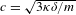is the sound speed (4 m s−1), V0 is the impact velocity, and σ = M/d is the linear mass density. The step shape suggests a convenient strategy for mimicking the sphere. Namely, for an appropriate value of κ, the average forces can nearly match up (Supplementary Fig. 5b). To find this value, we first run impacts at a fixed value of κ for several different drop heights (spread out over our experimental range of approximately 400 μm to 20 cm) and calculate the sum of the squared residuals between the average force and the prediction from Hertz. Calculating this quantity for different values of κ reveals a clear optimum (Supplementary Fig. 5c), which produces an Fav versus Hi curve that nicely approximates the experimental results (Supplementary Fig. 5b). Advantageously, this procedure ensures that the contact times for the experiments and simulations are similar (Supplementary Fig. 5d). For the data presented in Fig. 4 we use N = 201, m = 8.7 × 10−5 kg, δ = 7.5 × 10−5 m, and κ = 6.0 × 10−1 N. The results start becoming independent of discretization for N larger than 50 (see Supplementary Fig. 6).

### Interaction with the hard surface.

For the interaction with the hard surface, fs, we use the penalty method and turn on a very stiff spring for the bottom mass once it passes below y = 0. Additionally, we use the finite-element method practice of incorporating a damping term on the bottommost mass when it is below y = 0 to stabilize the contact. Concretely, this force is. Here ks is a spring constant, βs is a damping coefficient, and θ(−y0) is the Heaviside function to reflect that this only turns on for y0 < 0. In order for the surface to be ‘hard’, it must be the case that ks 3κ/δ. The damping parameter, βs, should be just large enough to maintain contact each time the lowest mass passes zero (until it is pushed off the surface again by the growing pressure). Beyond these criteria, the exact values of ks and βs do not significantly alter the gross features of impact, although extremely large values unnecessarily slow down computation. For the data in the main text we use ks = 105κ/δ and βs = 500 Ns m−1.

### Evolving contact area.

We account for the evolving contact area of an impacting sphere in each step of our numerics as is done in Hertzian contact mechanics. Explicitly, we calculate the intersection area of a sphere of diameter d located at the centre of mass of the chain, Ycm, with the plane located at y = 0. This is given by A = π[(d/2)2 − (d/2 − Ycm)2]θ(d/2 − Ycm). The Heaviside function, θ(d/2 − Ycm), is present to satisfy the requirement that the contact area is zero if Ycm > d/2. Having an evolving contact area is not necessary for energy injection. However, an evolving contact: better approximates the experimental situation where the energy injected per cycle is larger near the middle of each impact than at the beginning or end; and produces a smooth curve by rendering the effect of the discrete total number of cycles less pronounced.

### Parameters of the pressure evolution.

To produce trapping in our 1D model, we initialize the pressure to P = 0 Pa and use the following evolution: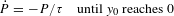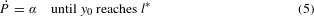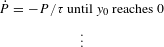As evident, this evolution depends on the values of the parameters l, α and τ, which we now estimate from our experiments.

First, we estimate a reasonable value for the length scale, l. This length must be smaller than, but on the order of, the gap height. Realistically, it will change for each cycle of a single impact owing to: the changing pre-compression of the sphere; and the changing contact area. It will also change from one impact to the next owing to differences in the dynamics as a function of the drop height. Practically, we use the zeroth-order approximation of a constant value because it is sufficient to recover the observed behaviours. We choose our estimate for the constant value based on observations of an equilibrium bounce and use l = 50 μm—on the order of maximum gap height seen in the middle of an impact for a sphere dropped from the equilibrium bounce height (Fig. 3b).

To estimate the value for the pressure build-up rate, α, we are guided by two calculations. First, as is evident in Fig. 3b, the gap under the sphere remains closed in the middle of the impact for Δt ≈ 0.25 ms. When the contact fully breaks, the upward force provided by the trapped vapour must be at least as large as the downward force from the Hertzian compression above. For a drop height of 3.5 cm, the Hertzian force from the compressed sphere above in the middle of the impact, Fmax, is on the order of 1 N (Supplementary Fig. 3c). With the aid of Hertzian theory or from our experimental data (for example, Supplementary Movies 3 and 4), we also know that the Hertzian contact area is Amax ≈ 10−4 m2. This gives the lower bound for the estimate, αFmax/AmaxΔt ≈ 4 × 107 Pa s−1.

As a second independent calculation, we use the data for the mass loss in combination with the ideal gas law. Again considering a drop from the steady bounce height, the mass lost is 1.5 × 102 μg per impact (Fig. 2d). Assuming 10 gap oscillations per impact and additional evaporative losses when the trap is open, we roughly estimate that the amount of vapour trapped during one cycle is on the order of 10 μg, which amounts to n = 6 × 10−7 mol. This is contained in a volume that scales like Amaxl. The time it takes for this to develop is, once again, Δt ≈ 0.25 ms. Using the ideal gas law, we therefore have α = nRT/AmaxlΔt ≈ 2 × 108 Pa s−1 (R is the universal gas constant and T ≈ 500 K is the temperature of the surface). This value is higher than our other estimate, and we expect this arises because the trapping process is not perfect—gas often escapes laterally in the middle of a cycle, thus rendering it less efficient. This is evidenced by the initially choppy development of the gap oscillations (Supplementary Movie 4). For the simulation data in Fig. 4, we use a value between our two estimates: α = 1.5 × 108 Pa s−1.

Finally, to inform our decision on a reasonable value for the time constant, τ, we consider the situation of pressure-driven evacuation of viscous vapour from a fixed gap (height l) trapped between two flat discs (area Amax). Accounting for Poiseuille’s flow and mass conservation, one can show that this system obeys Darcy’s law17,38 with a timescale given by τ = 12ηAmaxl2ΔP, where, η is the viscosity of the vapour (2 × 10−5 Pa s) and ΔP is the pressure difference between the centre and the edge (ΔPFmax/Amax ≈ 10 kPa). This gives a timescale of 10−4 s, which should be considered an upper bound for two reasons. First, the gap opens to heights greater than l, which further reduces the escape time. Second, the increasing volume of the gap itself reduces the pressure via the ideal gas law. In the simulations we use a slightly smaller value of τ = 5 × 10−5 s. Our model is not terribly sensitive to this parameter so long as it is not significantly larger than our upper bound—energy injection still occurs for infinitesimal values of τ as long as the lengthscale, l, and the pressure build-up parameter, α, are greater than zero.

### Data availability.

The data that support the plots within this paper and other findings of this study are available from the corresponding author upon request.